Home | | Business Maths 12th Std | Methods of finding initial Basic Feasible Solutions: Least Cost Method (LCM)

# Methods of finding initial Basic Feasible Solutions: Least Cost Method (LCM)

Operations Research: Transportation Problem: Methods of finding initial Basic Feasible Solutions: Least Cost Method (LCM)

Methods of finding initial Basic Feasible Solutions

There are several methods available to obtain an initial basic feasible solution of a transportation problem. We discuss here only the following three. For finding the initial basic feasible solution total supply must be equal to total demand.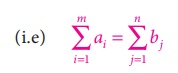## Method: Least Cost Method (LCM)

The least cost method is more economical than north-west corner rule,since it starts with a lower beginning cost. Various steps involved in this method are summarized as under.

Step 1: Find the cell with the least(minimum) cost in the transportation table.

Step 2: Allocate the maximum feasible quantity to this cell.

Step:3: Eliminate the row or column where an allocation is made.

Step:4: Repeat the above steps for the reduced transportation table until all the allocations are made.

Example 10.3

Obtain an initial basic feasible solution to the following transportation problem using least cost method.Here Oi and Dj denote ith origin and jth destination respectively.

Solution:

Total Supply = Total Demand = 24

Ōł┤ The given problem is a balanced transportation problem.

Hence there exists a feasible solution to the given problem.

Given Transportation Problem is:The least cost is 1 corresponds to the cells (O1, D1) and (O3, D4)

Take the Cell (O1, D1) arbitrarily.

Allocatemin (6,4) = 4 units to this cell.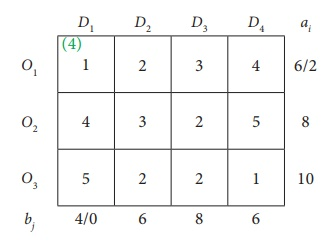The reduced table isThe least cost corresponds to the cell (O3, D4). Allocate min (10,6) = 6 units to this cell.The reduced table isThe least costis 2 corresponds to the cells (O1, D2), (O2, D3), (O3, D2), (O3, D3)

Allocate min (2,6) = 2 units to this cell.The reduced table is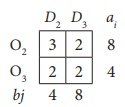The least cost is 2 corresponds to the cells (O2, D3), (O3, D2), (O3, D3)

Allocate min ( 8,8) = 8 units to this cell.The reduced table isHere allocate 4 units in the cell (O3, D2)Thus we have the following allocations:Transportation schedule :

O1ŌåÆ D1, O1ŌåÆD2, O2ŌåÆD3, O3ŌåÆD2, O3ŌåÆD4

Total transportation cost

=  (4├Ś1)+ (2├Ś2)+(8├Ś2)+(4├Ś2)+(6├Ś1)

=  4+4+16+8+6

=Rs. 38.

Example 10.4

Determine how much quantity should be stepped from factory to various destinations for the following transportation problem using the least cost method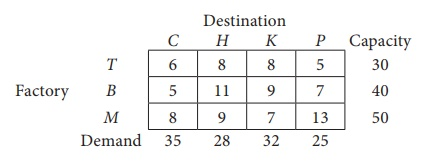Cost are expressed in terms of rupees per unit shipped.

Solution:

Total Capacity = Total Demand

Ōł┤ The given problem is balanced transportation problem.

Hence there exists a feasible solution to the given problem.

Given Transportation Problem isFirst Allocation:Second Allocation: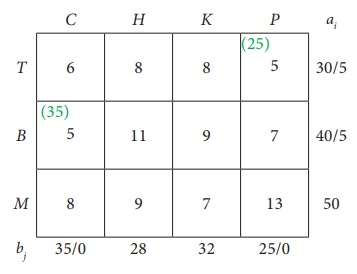Third Allocation:Fourth Allocation:Fifth Allocation:Sixth Allocation:Transportation schedule :

TŌåÆ H, TŌåÆP, BŌåÆC, BŌåÆH, MŌåÆH, MŌåÆK

The total Transportation cost = ( 5├Ś8) + (25├Ś5)+ (35├Ś5) + (5├Ś11)+ (18├Ś9) + (32├Ś7)

=  40+125+175+55+162+224

=  Ōé╣ 781

Tags : Solved Example Problems | Transportation Problem | Operations Research , 12th Business Maths and Statistics : Chapter 10 : Operations Research
Study Material, Lecturing Notes, Assignment, Reference, Wiki description explanation, brief detail
12th Business Maths and Statistics : Chapter 10 : Operations Research : Methods of finding initial Basic Feasible Solutions: Least Cost Method (LCM) | Solved Example Problems | Transportation Problem | Operations Research